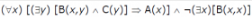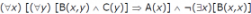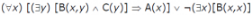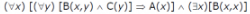Courses

# Consider the following logic program P A(x) <- B(x, y), C(y) <- B(x,x) Which of the following first order sentences is equivalent to P?a)b)c)d)Correct answer is option 'C'. Can you explain this answer? Related Test: Propositional And First Order Logic MCQ - 2

## Can you answer this question?

People are searching for an answer to this question.